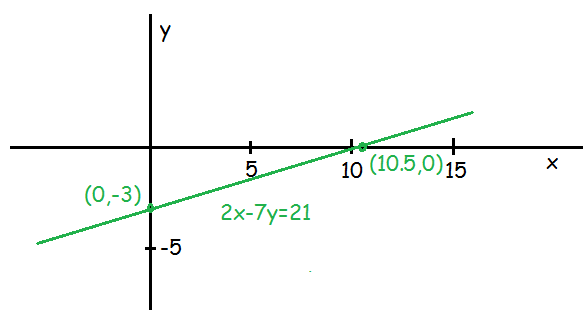When you tutor math, you know that everyone from grade 9 up needs to know this.

Suppose you need to graph 2x – 7y = 21. There are two basic methods: the slope-y intercept method, and the intercepts method. Today, we will use the intercepts method: it’s quicker.

Example: Graph 2x – 7y = 21

Solution:

We need to make a quick table of values, which we get by inserting 0 in one variable, then solving for the other. First, let’s “plug in” zero for x:

2(0) – 7y =21

Since 2(0) is just zero, we arrive at

-7y=21

Dividing both sides by -7 to find y, we get

y=-3

So, when x=0, y=-3. We enter that result in our table:

 x y 0 -3

Next, we ask about when y=0. Therefore, we plug 0 in for y as follows:

2x – 7(0) = 21

Once again: 7(0) is just zero, so we continue:

2x=21

Dividing by 2 on both sides, we arrive at

x=10.5

So, when y=0, x=10.5. We write that result in our table:

 x y 0 -3 10.5 0

Now it’s time to graph the result. From the table, we know that (0,-3) and (10.5,0) are points on our line. For a brush-up on how to graph points, see my post here. What we basically need to know is that to graph a point, we start from the origin (the center point), then go across the first number, up (or down) the second, and mark the point.

Once we’ve got the two points marked, we draw a line through them both. Like this:Look for more coverage on graphing lines in future posts:)

Jack of Oracle Tutoring by Jack and Diane, Campbell River, BC.

Tagged with: , ,